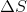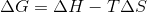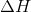High School Chemistry : Help with Entropy

Example Questions

Example Question #1 : Help With Entropy

Which of the following phases and states has the highest entropy?

Colloid

Aqueous solution

Liquid

Solid

Gas

Gas

Explanation:

Entropy is defined as the amount of disorder in a system and is favored in biological and chemical systems. Any system will prefer to have higher entropy, and spontaneous reactions will generally increase entropy in the system.

Gas particles move at higher velocity and with greater range than particles in liquids and solids. This contributes to their high level of entropy. Aqueous solutions gain entropy with the number of ions in solution, but do not reach the same level of entropy of gases. Colloids are homogenized mixtures, such as milk, and follow relatively the same principles as aqueous solutions.

Example Question #2 : Help With Entropy

Which of the following results in a decrease in entropy?

Melting ice

Breaking a mirror

Allowing a gas to diffuse

Explanation:

Entropy can be thought of as the tendency for a system to favor disorder. This means that the least ordered scenario in a system is typically favored by probability. Entropy increases when disorder is increased. Examples include an ice cube melting into a puddle, a gas diffusing all throughout a room, and a mirror shattering. Each of these either increases the energy of the system or results in the creation of multiple pieces/particles from a single object.

When building a road, materials are placed in an ordered, specific manner. This gives it a negative entropy.

Example Question #3 : Help With Entropy

For any chemical reaction __________.

the system experiences an increase in entropy

the universe loses entropy

the entropy of the surroundings must decrease

the entropy change of the universe is greater than or equal to zero

the entropy change of the universe is greater than or equal to zero

Explanation:

Any reaction can be thought of as taking place in a system, while the surroundings are the rest of the universe. It helps to remember that the entropy of the universe is constantly increasing. This means that the entropy change in the universe increases following every reaction. A system can have a decrease in entropy, as long as the entropy of the surroundings increases by a greater value.

Example Question #1 : Help With Entropy

Is a process more or less likely to occur as temperature increases whenis positive?

The answer cannot be determined without knowing the change in enthalpy

More likely because Gibbs free energy will increase

More likely because Gibbs free energy will decrease

Less likely because Gibbs free energy will increase

Less likely because Gibbs free energy will decrease

More likely because Gibbs free energy will decrease

Explanation:

Spontaneity, or the likelihood that a reaction will occur, is determined by Gibbs free energy. The equation for Gibbs free energy is:is the term for entropy and a negative value for Gibbs free energy indiciates a spontaneous reaction. Thus, as temperature increases the effective value of the entropy term increases as well (sinceis positive). Since the enthalpy,, remains constant, increasing the entropy term will have the total effect of decreasing the Gibbs free energy since entropy is subtracted from enthalpy. Decreasing the Gibbs free energy will result in a more spontaneous reaction.

All High School Chemistry Resources